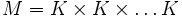# Base of a wreath product implies right-transitively 2-subnormal

This article describes a computation relating the result of the Composition operator (?) on two known subgroup properties (i.e., 2-subnormal subgroup (?) and Base of a wreath product (?)), to another known subgroup property (i.e., 2-subnormal subgroup (?))
View a complete list of composition computations
This article gives the statement and possibly, proof, of an implication relation between two subgroup properties. That is, it states that every subgroup satisfying the first subgroup property (i.e., base of a wreath product) must also satisfy the second subgroup property (i.e., right-transitively 2-subnormal subgroup)
View all subgroup property implications | View all subgroup property non-implications
Get more facts about base of a wreath product|Get more facts about right-transitively 2-subnormal subgroup

## Statement

### Property-theoretic statement

The statement has the following equivalent forms:

1. The subgroup property of being the base of a wreath product is stronger than the subgroup property of being a right-transitively 2-subnormal subgroup.
2. The composition of the subgroup property of being 2-subnormal with the subgroup property of being the base of a wreath product implies the subgroup property of being 2-subnormal.

2-subnormal$*$ Base of a wreath product$\le$ 2-subnormal

### Verbal statement

1. Any base of a wreath product in a group is a right-transitively 2-subnormal subgroup.
2. Any 2-subnormal subgroup of the base of a wreath product is 2-subnormal in the whole group.

### Statement with symbols

1. If$K$ is the base of a wreath product in$G$, then$K$ is a right-transitively 2-subnormal subgroup of$G$.
2. If$H$ is a 2-subnormal subgroup of$K$ and$K$ is the base of a wreath product in$G$, then$H$ is 2-subnormal in$G$.

## Facts used

1. Direct factor implies transitively normal: Any normal subgroup of a direct factor is normal.

## Proof

Given: A group$G$ with$K$ the base of a wreath product. In other words,$G = (K \times K \times \dots K) \rtimes L$ (the direct product may be infinite), and the subgroup$K$ is the first direct factor.$H$ is a 2-subnormal subgroup of$K$. Let$M = K \times K \times \dots K$.

To prove:$H$ is 2-subnormal in$G$.

Proof: Let$R$ be the normal closure of$H$ in$K$.

1. (Fact used: Fact (1), direct factor implies transitively normal):$R$ is normal in$K$, and$K$ is a direct factor of$M$. Thus,$R$ is normal in$M$.
2. (Given data used:$G$ is a wreath product of$K$ and$L$): Since$R$ is normal in$M$, the normal closure$S$ of$R$ in$G$ is given as the closure of$R$ under the action of$L$. This is a direct product of isomorphic copies of$R$ for all the coordinates in the orbit of the first coordinate.
3. (Given data used:$H$ is 2-subnormal in$K$): Since$H$ is 2-subnormal in$K$,$H$ is normal in its normal closure$R$ in$K$.
4. (Fact used: Fact (1), direct factor implies transitively normal):$H$ is normal in$R$, and$R$ is a direct factor of$S$, so$H$ is normal in$S$.
5. Since$H$ is normal in$S$, and$S$ is normal in$G$ ($S$ is, after all, defined as the normal closure of$R$ in$G$),$H$ is 2-subnormal in$G$.